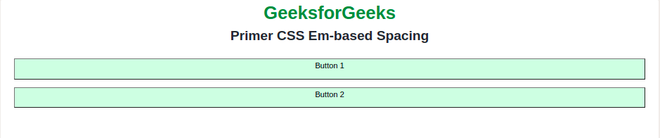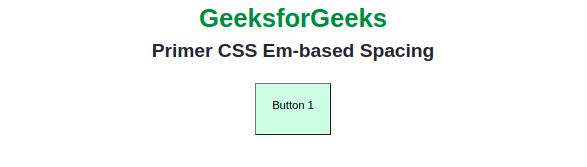Open in App
Not now

# Primer CSS Em-based Spacing

• Last Updated : 18 Nov, 2022

Primer CSS Spacing contains Em-based Spacing which is mainly used to provide the spacing between the components, having the values in em, for which the value combined with typography, line height, or the total height becomes sensible numbers.

GitHub’s body font size is 14px which is difficult to work with, so we sometimes can’t achieve a whole number. Variables listed below are preferred for use within components and custom CSS.

Syntax:

`\$name-of-the-variable: value;`

Below examples illustrate the Primer CSS Em-based Spacing

Example 1: In this example, we will render two buttons, by creating our own variable \$em-spacer-12 that will hold the value of 1em.

## HTML

 ` ` `<``html` `lang``=``"en"``> ` ` `  `<``head``> ` `    ``<``meta` `charset``=``"UTF-8"``> ` `    ``<``meta` `http-equiv``=``"X-UA-Compatible"` `content``=``"IE=edge"``> ` `    ``<``meta` `name``=``"viewport"` `content``=``"width=device-width, initial-scale=1.0"``> ` `    ``<``link` `href``= ` `"https://unpkg.com/@primer/css@^20.2.4/dist/primer.css"` `rel``=``"stylesheet"` `/> ` ` `  ` ` ` `  `<``body``> ` `    ``<``div` `class``=``"text-center"``> ` `        ``<``h1` `class``=``"color-fg-success"``> ` `            ``GeeksforGeeks ` `        `` ` `        ``<``h2``>Primer CSS Em-based Spacing ` `        ``<``br``> ` `        ``<``div` `class``=``"gap mx-4"``> ` `            ``<``button` `class``=``"color-bg-success"``> ` `                ``<``p``>Button 1 ` `            `` ` `            ``<``button` `class``=``"color-bg-success"``> ` `                ``<``p``>Button 2 ` `            `` ` `        `` ` `    `` ` ` ` ` `  ``

SCSS:

```\$em-spacer-12: 1em;
.gap {
gap:\$em-spacer-12;
}```

CSS:

```.gap{
gap: 1em;
}```

Output:Example 2: In this example, we will use spacing inside of the button element as padding.

## HTML

 ` ` `<``html` `lang``=``"en"``> ` ` `  `<``head``> ` `    ``<``meta` `charset``=``"UTF-8"``> ` `    ``<``meta` `http-equiv``=``"X-UA-Compatible"` `content``=``"IE=edge"``> ` `    ``<``meta` `name``=``"viewport"` `content``=``"width=device-width, initial-scale=1.0"``> ` `    ``<``link` `href``= ` `"https://unpkg.com/@primer/css@^20.2.4/dist/primer.css"` `rel``=``"stylesheet"` `/> ` ` ` ` `  `<``body``> ` `    ``<``div` `class``=``"text-center"``> ` `        ``<``h1` `class``=``"color-fg-success"``> ` `            ``GeeksforGeeks ` `        `` ` `        ``<``h2``>Primer CSS Em-based Spacing ` `        ``<``br``> ` `        ``<``div` `class``=``"mx-4"``> ` `            ``<``button` `class``=``"color-bg-success"``> ` `                ``<``p` `class``=``"left"``>Button 1 ` `            `` ` `        `` ` `    `` ` ` ` ` `  ``

SCSS:

```\$em-spacer-12: 1em;
.left {
}```

CSS:

```.left{
}```

Output:References: https://primer.style/css/support/spacing

My Personal Notes arrow_drop_up
Related Articles